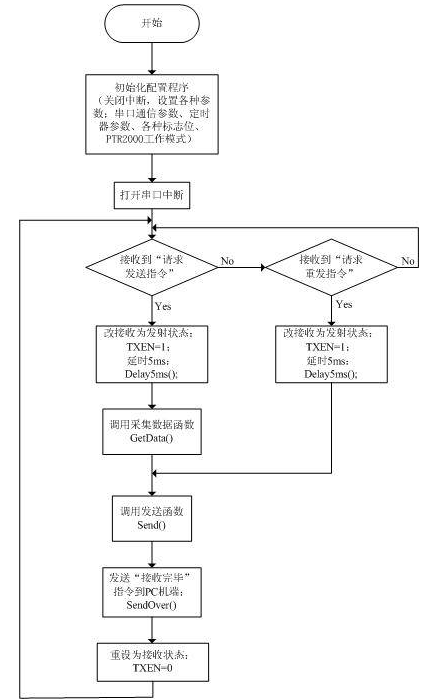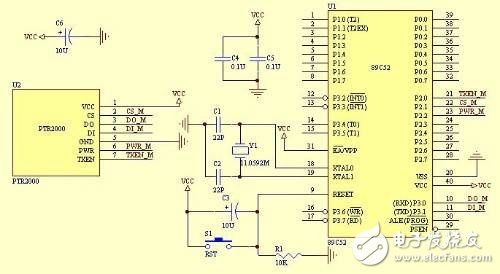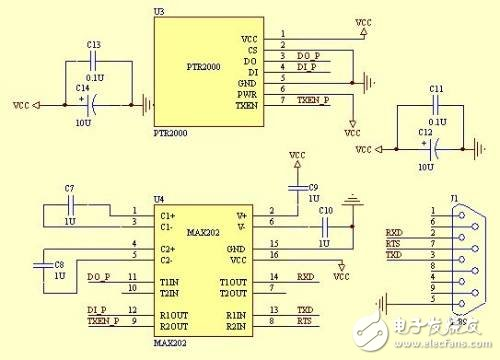# AT89C52单片机与PC机之间的无线数据传输设计

+关注

1、 单片机端：AT89C52单片机芯片，用于控制无线模块的发射和接受;PTR2000无线数据传输模块，使用了433MHz IGM频段，是真正的单片UHF无线收发一体芯片，可以和单片机的串口直接相连。

2、 PC端：PTR2000无线数据传输模块;TTL电平转换RS-232电平芯片MAX202。PC端：//wireless.h程序

#ifndef _WIRELESS_H // 防止wireless.h被重复引用

#define _WIRELESS_H

#include // 引用标准库的头文件

#include

#define uchar unsigned char

#define uint unsigned int

#define REQ_SEND 0x33

#define REQ_RESEND 0x66

#define SEND_OVER 0x99

//为简化起见，假设了7位固定的采集数据

#define DATA0 0x10

#define DATA1 0x20

#define DATA2 0x30

#define DATA3 0x40

#define DATA4 0x50

#define DATA5 0x60

#define DATA6 0x70

sbit TXEN = P2^0;

sbit CS = P2^1;

sbit PWR = P2^2;

void ClearT_buf（）; // 清除t_buf函数

void ClearR_buf（）; // 清除r_buf函数

void Delay5ms（）; // 延时5ms

void GetData（）; // 采集数据

void Send（）; // 采集并发送函数

void SendOver（）; // 通知PC机发送结束函数

xdata uchar Flag_StartRec = 0 ; //开始接受数据标志位

xdata uchar Flag_ReqSend = 0 ; //请求发送标志位

xdata uchar Flag_ReqReSend = 0 ; //请求重发标志位

xdata uchar Flag_Delay5ms = 1 ; //延时5ms标志

xdata uchar Data［7］; //采集的7个字节数据

xdata uchar tCount = 0 ;

xdata uchar t_buf［11］; // 1开始字节“\$”，1长度字节LEN，

// 7字节数据，1校验和字节，

// 1 结束字节“*”

xdata uchar rCount = 0 ;

xdata uchar r_buf［5］; // 1开始字节“\$”，1长度字节LEN，

// 1字节指令，1校验和字节，

// 1 结束字节“*”

#endif

//wireless.c程序

#include “wireless.h”

/* 定时器0中断服务子程序 */

void timer（） interrupt 1 using 2

{

Flag_Delay5ms = 0;

TH0 = -5000/256;

TL0 = -5000%256;

}

void main（void）

{

ClearT_buf（）;

ClearR_buf（）;

TXEN = 0; //初始为接收状态

PWR = 1; //正常工作模式

CS = 0; //选择频道0

EA=0;

/* 11.0592MHz下，设置串行口9600波特率，工作方式1

8位数据位，1位停止位，无奇偶校验，*/

TMOD = 0x21; //定时器1工作模式1，定时器0工作模式2

SCON = 0x50; //串行口工作方式1，REN=1

PCON = 0x00; //SMOD=0

TL1 = 0xfd;

TH1 = 0xfd;

TR1=1; //定时器1开始计时

PT0 = 1; //定时器0高优先级

TH0 = -5000/256; //5ms

TL0 = -5000%256;

IE = 0x90; //EA=1，ES=1：打开串口中断

while （1）

{

if （Flag_ReqSend） //收到“请求发送”指令

{

TXEN = 1; //改变为发射状态

Delay5ms（）; //延时5ms

GetData（）; //采集数据

Send（）; //发送

Flag_ReqSend = 0;

SendOver（）; //通知PC机发送结束

TXEN = 0; //重设为接收状态

}

else if （Flag_ReqReSend） //收到“请求重发”指令

{

TXEN = 1; //改变为发射状态

Delay5ms（）; //延时5ms

Send（）; //重发

Flag_ReqReSend = 0;

SendOver（）; //通知PC机发送结束

TXEN = 0; //重设为接收状态

}

}

}

/* 串口中断服务子程序 */

void serial （ ） interrupt 4 using 1

{

RI = 0 ;

/* 判断是否收到字符‘\$’，其数值为0x24，置开始接收标志位*/

if （（！Flag_StartRec） && （SBUF == 0x24））

{

Flag_StartRec = 1;

}

if （Flag_StartRec）

{

if （rCount 《5）

{

r_buf［rCount］ = SBUF;

rCount ++;

}

/* 判断是否收到字符‘*’，其数值为0x2A，根据接收的指令设置相应标志位*/

if （（r_buf［rCount -1］ == 0x2A）||（rCount == 5））

{

rCount = 0;

Flag_StartRec = 0;

if （r_buf［2］ == REQ_SEND） //收到“请求发送”指令

{

Flag_ReqSend = 1;

}

if （r_buf［2］ == REQ_RESEND） //收到“请求重发”指令

{

Flag_ReqReSend = 1;

}

}

else

ClearR_buf（）;

}

}

void ClearT_buf（void）

{

uchar xdata k ;

for （k=0;k++;k《11）

{

t_buf［k］ = 0;

}

}

void ClearR_buf（void）

{

uchar xdata k ;

for （k=0;k++;k《5）

{

r_buf［k］ = 0;

}

}

void Delay5ms（void）

{

TR0=1;

ET0=1;

while（ Flag_Delay5ms）;

ET0 = 0;

TR0 = 0;

Flag_Delay5ms = 1;

}

//采集数据函数经过简化处理，取固定的7个字节数据

void GetData（void）

{

Data［0］=DATA0;

Data［1］=DATA1;

Data［2］=DATA2;

Data［3］=DATA3;

Data［4］=DATA4;

Data［5］=DATA5;

Data［6］=DATA6;

}

//单片机端发送数据函数

void Send（void）

{

uchar xdata j = 0;

uchar xdata len = 0;

uchar xdata CheckSum = 0 ;

t_buf［0］=0x24; //起始位

t_buf［1］=0x07; //7个数据字节

len=t_buf［1］;

CheckSum = CheckSum + len;

for （ j=0;j++;j

{

t_buf［j+2］ = Data［j］;

CheckSum = CheckSum + t_buf［j+2］;

}

t_buf［9］ = CheckSum; //校验和字节

t_buf［10］ = 0x2A; //停止位

for （j=0;j++;j《11）

{

TI =0 ;

SBUF = t_buf［j］;

while （ TI ==0 ）;

TI =0 ;

}

}

//通知PC机端发送结束函数

void SendOver（void）

{

TI =0 ;

SBUF = 0x24;

while （ TI ==0 ）;

TI =0 ;

SBUF = 0x01;

while （ TI ==0 ）;

TI =0 ;

SBUF = SEND_OVER; //通知PC机端“发送结束”

while （ TI ==0 ）;

TI =0 ;

SBUF = 0x99; //校验和字节

while （ TI ==0 ）;

TI =0 ;

SBUF = 0x2A;

while （ TI ==0 ）;

TI =0 ;

}